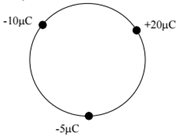# Problem: Three point charges are held on the circumference of a circle of radius 20 cm as shown in the figure. Assume that the electric potential is defined to be zero at infinity. Determine the electric potential at the center of the circle. (a) +6.0 x 104 V (b) +2.3 x 105 V (c) -4.6 x 105 V (d) –7.8 x 105 V (e) zero volts

###### FREE Expert Solution
99% (374 ratings)
###### Problem Details

Three point charges are held on the circumference of a circle of radius 20 cm as shown in the figure. Assume that the electric potential is defined to be zero at infinity. Determine the electric potential at the center of the circle.

(a) +6.0 x 10V

(b) +2.3 x 10V

(c) -4.6 x 10V

(d) –7.8 x 10V

(e) zero voltsFrequently Asked Questions

What scientific concept do you need to know in order to solve this problem?

Our tutors have indicated that to solve this problem you will need to apply the Electric Potential concept. You can view video lessons to learn Electric Potential. Or if you need more Electric Potential practice, you can also practice Electric Potential practice problems.

How long does this problem take to solve?

Our expert Physics tutor, Juan took 3 minutes and 38 seconds to solve this problem. You can follow their steps in the video explanation above.

What professor is this problem relevant for?

Based on our data, we think this problem is relevant for Professor Schulte's class at UCF.